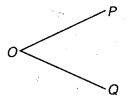# Class 6 Maths NCERT Solutions for Chapter 4 Basic Geometrical Ideas Ex – 4.1

## Basic Geometrical Ideas

Question 1.
Use the figure to name:

(a) Five points
(b) A line
(c) Four rays
(d) Five line segments

Solution:
Clearly, from the given figure

(a)
5 points are points, O, C, B, D and E.
(b) A line is [altex]\overleftrightarrow { BD } [/latex]
(c) 4 rays are$\overleftrightarrow { OC } ,\overleftrightarrow { OB } ,\overleftrightarrow { OD }$ and$\overleftrightarrow { ED }$.
(d) 5 line segments are$\overline { OE } ,\overline { OD } ,\overline { DE, } \overline { OB }$ and$\overline { BD }$.

Question 2.
Name the line given in all possible (twelve) ways, choosing only two letters at a time from the four given.Solution:
All the possible ways of naming the given by choosing only two letters at a time out of 4 letters are$\overleftrightarrow { AB } ,\overleftrightarrow { AC } ,\overleftrightarrow { AD } ,\overleftrightarrow { BC } ,\overleftrightarrow { BD } ,\overleftrightarrow { CD } ,\overleftrightarrow { DC } ,\overleftrightarrow { DB } ,\overleftrightarrow { CB } ,\overleftrightarrow { DA } ,\overleftrightarrow { CA }$ and$\overleftrightarrow { BA }$.

Question 3.
Use the figure to name :

(a) Line containing point E.
(b) Line passing through A.
(c) Line on which O lies.
(d) Two pairs of intersecting lines.Solution:
Clearly, from the given figure
(a) Line containing pqint E is$\overleftrightarrow { AE }$.
(b) Line passing through A is$\overleftrightarrow { AE }$.
(c) O lies on the line$\overleftrightarrow { CB }$.
(d) Two pairs of intersecting lines are$\overleftrightarrow { CO } ,\overleftrightarrow { AE }$ and$\overleftrightarrow { AE } ,\overleftrightarrow { EF }$.

Question 4.
How many lines can pass through

(a) one given point?
(b) two given points?

Solution:
(a)
An unlimited number of lines can be drawn passing through a given point.
(b) Exactly one line can be drawn passing through two different given points in a plane.

Question 5.
Draw a rough figure and label suitably in each of the following cases:

(a) Point P lies on$\overline { AB }$.
(b)$\overleftrightarrow { XY }$ and$\overleftrightarrow { PQ }$ intersect at M.
(c) Line l contains E and F but not D.
(d)$\overleftrightarrow { OP }$ and$\overleftrightarrow { OQ }$ meet at O.

Solution:
A rough figure and labelled suitably for the given cases are as under:
(a) Point P lies on$\overleftrightarrow { AB }$ :

(b)$\overleftrightarrow { XY }$ and$\overleftrightarrow { PQ }$ intersect at M:

(c)
Line l contains E and F but not D:

(d)$\overleftrightarrow { OP }$ and$\overleftrightarrow { OQ }$ meet at O.Question 6.
Consider the following figure of line MN. Say whether following statements are true or false in context of the given figure.

(a)
Q, M, O, N, P are points on the line$\overleftrightarrow { MN }$ .
(b) M, O, N are points on a line segment$\overline { MN }$.
(c) M and N are end points of line segment$\overline { MN }$.
(d) O and N are end points of line segment$\overline { OP }$.
(e) M is one of the end points of line segment$\overline { QO }$.
(f) M is point on ray$\overrightarrow { OP }$.
(g) Ray$\overrightarrow { OP }$ is different from ray$\overrightarrow { QP }$.
(h) Ray$\overrightarrow { OP }$ is same as ray$\overrightarrow { OM }$.
(i) Ray$\overrightarrow { OM }$ is not opposite to ray$\overrightarrow { OP }$.
(j) O is not an initial point of$\overrightarrow { OP }$.
(k) N is the initial point of$\overrightarrow { NP }$ and$\overrightarrow { NM }$.

Solution:
In the context of the given figure, the given statement is:
(a) True
(b) True
(c) True
(d) False
(e) False
(f) False
(g) True
(h) False
(i) True
(j) False
(k) True